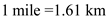# Many highways have a speed limit of 55 mi/h. (a) Is this speed greater than, less than, or equal to 55 km/h?

Many highways have a speed limit of 55 mi/h. (a) Is this speed greater than, less than, or equal to 55 km/h? Explain. (b) Find the speed limit in km/h that corresponds to 55 mi/h.

CONCEPT:
The relation between mile and kilometer is given as follows.(a) The speed of 55 mi/h is compared with 55 km/h as follows.
Convert the speed of 1 mi/hinto the units of km/h.The equivalent speed of 1 mi/h in the units of km/h is 1.61 km/h.As the speed of 1 mi/h is greater than 1 km/h, it is clear that the speed limit of 55 mi/h is greater than 55 km/h.

(b) The speed limit in km/h corresponding to 55 mi/h is calculated as follows.
Convert the speed of 55 mi/h into the units of km/h.Therefore, the speed limit in km/h corresponding to 55 mi/h is 88.6 km/h.
The speed limit in km/h is 88.6 km/h. corresponding to the speed of 55 mi/h.